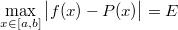# Remez tutorial 1/5: exp(x) the quick way

This is a hands-on example of the Lol Remez toolkit.

In this section we are going to approximate the exp(x) function on [-1,1] using a polynomial.

## Getting started

If you do not have the full Lol Engine source code, download and unpack the latest LolRemez tarball.

The file you should edit is remez.cpp.

## What does Remez do?

Given a function f and a range [a,b], the Remez algorithm looks for the polynomial P(x) that minimises the following error value E:Note that E is not a parameter. It is a value that the algorithm computes together with the polynomial. Though we will see ways to fine-tune the error, a general rule is: if you want a smaller error, ask for a polynomial of higher degree.

## Source code

#include "lol/math/real.h"
#include "lol/math/remez.h"

using lol::real;
using lol::RemezSolver;

real f(real const &x) { return exp(x); }

int main(int argc, char **argv)
{
RemezSolver<4, real> solver;
solver.Run(-1, 1, f, 40);
return 0;
}


What does this mean?

• We declare function f which returns the exponential of x: this is the function we want to approximate.
• We create a RemezSolver object for 4th-degree polynomials and real numbers. As of now, no other number types are supported.
• We run the solver on the [-1,1] range, approximating function f to 40 decimals of precision. The larger the precision, the more iterations are necessary, but the process usually takes only a few seconds for small functions.

## Compilation

If you are using LolRemez, just put the above source code in remez.cpp and type:

make


## Execution

To launch the test, type:

./remez


After all the iterations the output should be as follows:

Step 7 error: 5.466676005137979474524666548947155992203e-4
Polynomial estimate:
x**0*1.000090000102127639946253082819502265543
+x**1*9.973092516744464320538318907902496576588e-1
+x**2*4.988351170902359155314941477995868737492e-1
+x**3*1.773452743688412268810974931504564418976e-1
+x**4*4.415551762288022300015839013797254330891e-2


## Using the results

The above results can be used in a more CPU-friendly implementation such as the following one:

double fastexp(double x)
{
const double a0 = 1.000090000102127639946253082819502265543;
const double a1 = 9.973092516744464320538318907902496576588e-1;
const double a2 = 4.988351170902359155314941477995868737492e-1;
const double a3 = 1.773452743688412268810974931504564418976e-1;
const double a4 = 4.415551762288022300015839013797254330891e-2;

return a0 + x * (a1 + x * (a2 + x * (a3 + x * a4)));
}


## Analysing the results

Plotting the real exponential function and our fastexp function gives the following curves:The curves are undistinguishable. Actually they differ by no more than 5.46668e-4, which is the value the ./remez output gave.

It can be verified on the following error curve:## Conclusion

You should now be all set up for your own minimax polynomial computation!

Please report any trouble you may have had with this document to sam@hocevar.net. You may then carry on to the next section: switching to relative error.